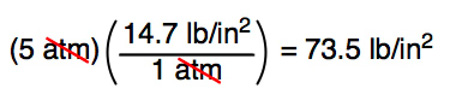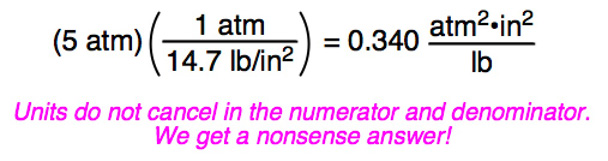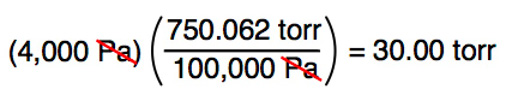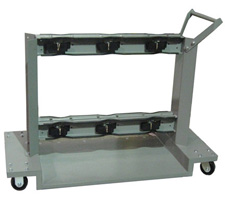MSDSTopics Free Sites FAQ's Regulations Glossary Software Suppliers Books Forum Poll Fun stuff Quiz Store Understand your MSDS with the MS-Demystifier Search ALL our MSDS info

Pressure Unit Conversions

Definition

Pressure is a measure of the force against a surface. Pressure is usually expressed as a force per unit area.

Here is a handy conversion calculator for some common pressure terms. The definitions of each term are in the following section.

 Pressure Conversion Calculator(Enter number on the left side; answer appears on the right side) This many: pounds per square inchatmospherestorrbarmillibarpascalskilopascalsmegapascalsgigapascals Equals pounds per square inchatmospherestorrbarmillibarpascalskilopascalsmegapascalsgigapascals

Many of the items you will find on an MSDS come in both English (U.S. Customary System) and metric (International System or SI or cgs) units. The metric system has been adopted by almost every country except the United States. Even in the U.S., scientists and technical people use the metric system because of its ease of use.

If you are unfamiliar with the terms used in these definitions, see our mass unit and distance unit conversion pages.

Pounds per square inch
(psi, PSI, lb/in2, lb/sq in)
Commonly used in the U.S., but not elsewhere. Normal atmospheric pressure is 14.7 psi, which means that a column of air one square inch in area rising from the Earth's atmosphere to space weighs 14.7 pounds.
Atmosphere
(atm)
Normal atmospheric pressure is defined as 1 atmosphere. 1 atm = 14.6956 psi = 760 torr.
Torr
(torr)
Based on the original Torricelli barometer design, one atmosphere of pressure will force the column of mercury (Hg) in a mercury barometer to a height of 760 millimeters. A pressure that causes the Hg column to rise 1 millimeter is called a torr (you may still see the term 1 mm Hg used; this has been replaced by the torr. "mm Hg" is commonly used for blood pressure measurements). 1 atm = 760 torr = 14.7 psi.
Bar
(bar)
The bar nearly identical to the atmosphere unit. One bar = 750.062 torr = 0.9869 atm = 100,000 Pa.
Millibar
(mb or mbar)
There are 1,000 millibar in one bar. This unit is used by meteorologists who find it easier to refer to atmospheric pressures without using decimals. One millibar = 0.001 bar = 0.750 torr = 100 Pa.
Pascal
(Pa)
1 pascal = a force of 1 Newton per square meter (1 Newton = the force required to accelerate 1 kilogram one meter per second per second = 1 kg.m/s2; this is actually quite logical for physicists and engineers, honest). 1 pascal = 10 dyne/cm2 = 0.01 mbar. 1 atm = 101,325 Pascals = 760 mm Hg = 760 torr = 14.7 psi.
Kilopascal
(kPa)
The prefix "kilo" means "1,000", so one kilopascal = 1,000 Pa. Therefore, 101.325 kPa = 1 atm = 760 torr and 100 kPa = 1 bar = 750 torr.
Megapascal
(MPa)
The prefix "mega" means "1,000,000", so one megapascal = 1,000 kPa = 1,000,000 Pa = 9.869 atm = 145 psi.
Gigapascal
(GPa)
The prefix "giga" means "1,000,000,000", so one gigapascal = 1,000 MPa = 1,000,000 kPa = 1,000,000,000 Pa = 9,870 atm = 10,000 bar. Pressures of several gigapascals can convert graphite to diamond or make hydrogen a metallic conductor! Such high pressures are rarely encountered in everyday life.

Some Worked Examples

CORRECT: Convert 5 atm to psi:WRONG: Convert 5 atm to psi:CORRECT: Convert 4,000 pascal to torr:Safety Emporium has gas cylinder signs, racks, carts, clamps, lockouts and more to ensure your workers' safety.

SDS Relevance

Be very careful to note the units when reading numbers on a Safety Data Sheet. If you ever perform a calculation of any sort, always remember to write the units next to each number in your calculation and make sure that they cancel properly. If they don't, your calculation was incorrect!